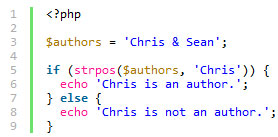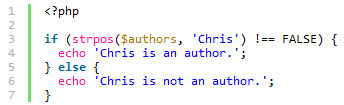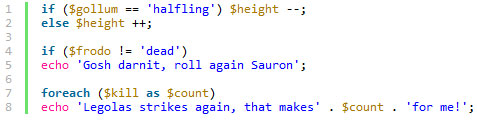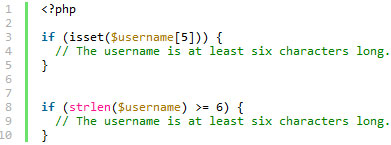# PHP开发的9条经验技巧

### 1.首要意识：安全

1. 永远不要相信用户输入的东西。（老话了，但这是真的）

2. 将自己需要输出的数据进行转义。

```SELECT FROM users WHERE username = \$_POST['username'] AND password = \$_POST['password'];
```

### 2.明确地知道各个比较操作符的差别

PHP的比较操作符,这其实可以说是一个很小的注意点，但是在某些时候真的很重要。比如说很多时候我们得考虑清楚，该用==还是===，如果你使用过strpos()这个函数，下面的代码可能会给你一个直观的感受：### 3.可以减少使用else就少使用else

```if( this condition ) { \$x = 5; } else{ \$x = 10;}
```

```\$x = 10;
if( this condition )
{ \$x = 5; }
```

### 4.去掉不必要的括号

```if (\$gollum == 'halfling') { \$height --; }
```

```if (\$gollum == 'halfling') \$height --;
```### 5.多用str_replace()

```str_replace()
ereg_replace()
preg_replace()
```

### 6.使用三元运算符

``` \$host = strlen(\$host) > 0 ? \$host : htmlentities(\$host);
```

### 9.用isset()代替strlen()• 暂无评论